# Fahrenheit and Celsius Conversion Formulas Fahrenheit to Celsius

• Slides: 7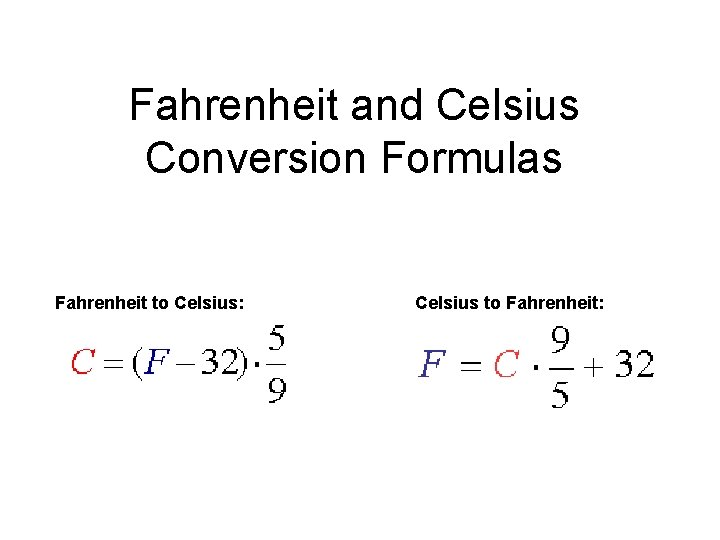Fahrenheit and Celsius Conversion Formulas Fahrenheit to Celsius: Celsius to Fahrenheit: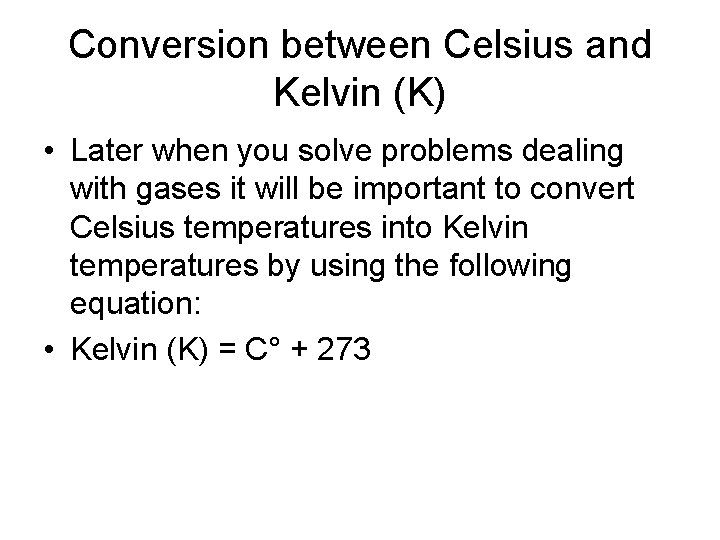Conversion between Celsius and Kelvin (K) • Later when you solve problems dealing with gases it will be important to convert Celsius temperatures into Kelvin temperatures by using the following equation: • Kelvin (K) = C° + 273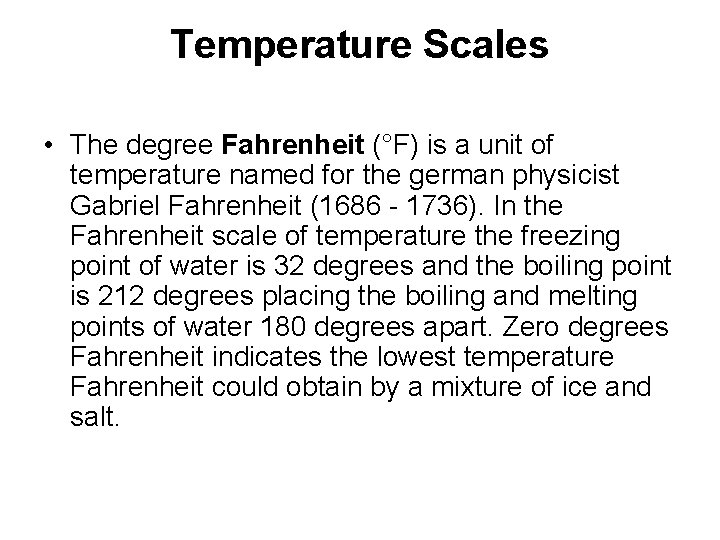Temperature Scales • The degree Fahrenheit (°F) is a unit of temperature named for the german physicist Gabriel Fahrenheit (1686 - 1736). In the Fahrenheit scale of temperature the freezing point of water is 32 degrees and the boiling point is 212 degrees placing the boiling and melting points of water 180 degrees apart. Zero degrees Fahrenheit indicates the lowest temperature Fahrenheit could obtain by a mixture of ice and salt.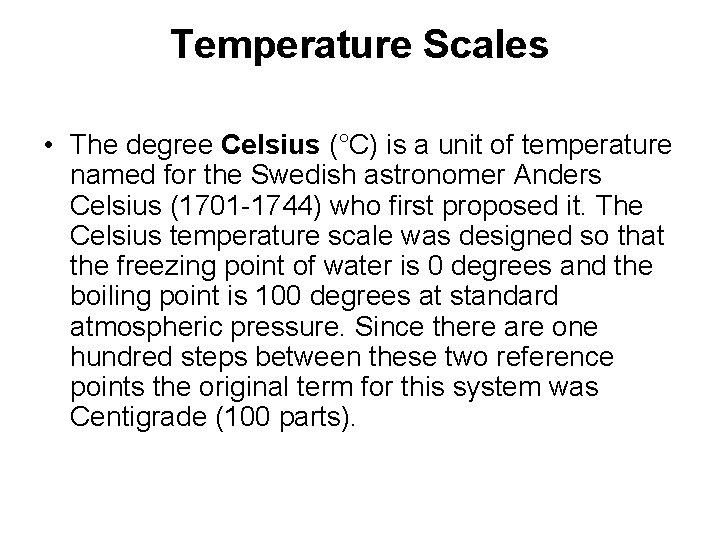Temperature Scales • The degree Celsius (°C) is a unit of temperature named for the Swedish astronomer Anders Celsius (1701 -1744) who first proposed it. The Celsius temperature scale was designed so that the freezing point of water is 0 degrees and the boiling point is 100 degrees at standard atmospheric pressure. Since there are one hundred steps between these two reference points the original term for this system was Centigrade (100 parts).Examples • How many degrees Celsius are 68 degrees Fahrenheit? Replace Fahrenheit with 68 and solve for Celsius: • C = (68 - 32) * 5/9, C = 36 * 5/9, C = 20 20 °C = 68 °F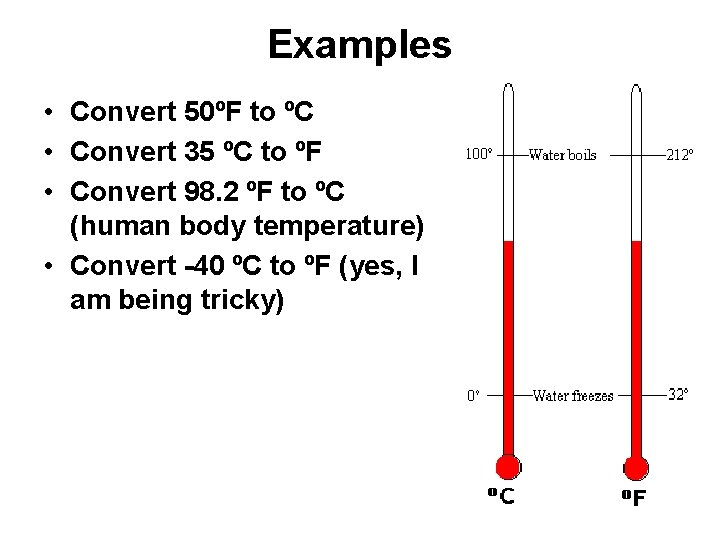Examples • Convert 50ºF to ºC • Convert 35 ºC to ºF • Convert 98. 2 ºF to ºC (human body temperature) • Convert -40 ºC to ºF (yes, I am being tricky)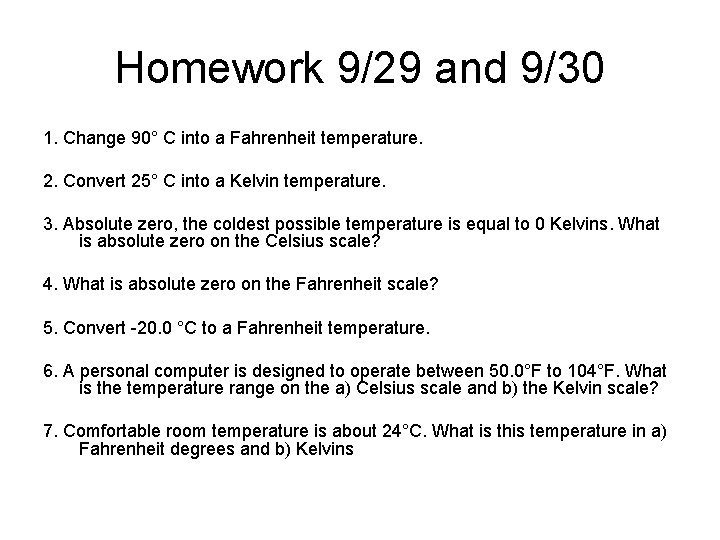Homework 9/29 and 9/30 1. Change 90° C into a Fahrenheit temperature. 2. Convert 25° C into a Kelvin temperature. 3. Absolute zero, the coldest possible temperature is equal to 0 Kelvins. What is absolute zero on the Celsius scale? 4. What is absolute zero on the Fahrenheit scale? 5. Convert -20. 0 °C to a Fahrenheit temperature. 6. A personal computer is designed to operate between 50. 0°F to 104°F. What is the temperature range on the a) Celsius scale and b) the Kelvin scale? 7. Comfortable room temperature is about 24°C. What is this temperature in a) Fahrenheit degrees and b) Kelvins